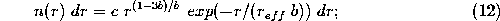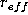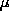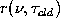Next: IR Optical Depth Inversion Up: Infrared Spectrum Previous: FASCOD3P Model

### IR Scattering from Mie Model

The infrared scattering cross-section for molecules is much smaller than the absorption cross-section; and extinction in the IR is dominated by absorption such that scattering can be neglected. However, ice crystals and water droplets found in clouds are of similar magnitude or greater in size than wavelengths associated with IR radiation. Although extinction by absorption continues to dominate, scattering of upwelling terrestrial and atmospheric emission from below the cloud will provide a small contribution to the downwelling measured radiance.

Model simulations using Mie theory and an adding-doubling model (Collard et al., 1995) suggest that the cirrus scattered signal received at the surface is at least an order of magnitude smaller than cloud emission. Up to this point, each term in the RTE can be determined using atmospheric state measurements. Invocation of Mie theory requires knowledge of cloud particle size to determine the phase function, such that a size distribution (Hansen, 1971) must be assumed,where n(r) is the number of particles of radius r and c is a normalization constant. The calculations use an effective radius,, of 50m and effective variance, b, of 0.25. A 50mis consistent with measured values for cirrus (Poellot and Henderson, 1994).

The resultant phase function derived from the Mie calculations is a function of the particle size distribution and the index of refraction. The spectral reflectance has an angular dependence described by the phase function and has a magnitude that is dependent on the concentration of particles. This implies that the reflectance will vary for each microwindow and depend upon the cloud infrared transmissivity, which cannot be inverted from the RTE until the reflectance is known. Therefore, an iterative solution is necessary, which requires parameterization of the reflectance as a function of cloud transmissivity,. The reflectance is determined with the adding-doubling model by monitoring the ratio of upwelling relative to downwelling radiant flux at the cloud base.

This approach is feasible if a particle size distribution is assumed and an independent parameterization is determined for each microwindow region. The latter constraint is necessary due to the frequency dependent index of refraction for ice. This technique is outlined for a sample AERI microwindow wavenumber, and the polynomial fit coefficients are tabulated, in Appendix D.Next: IR Optical Depth Inversion Up: Infrared Spectrum Previous: FASCOD3P Model

Daniel DeSlover
Sun Aug 11 10:02:40 CDT 1996